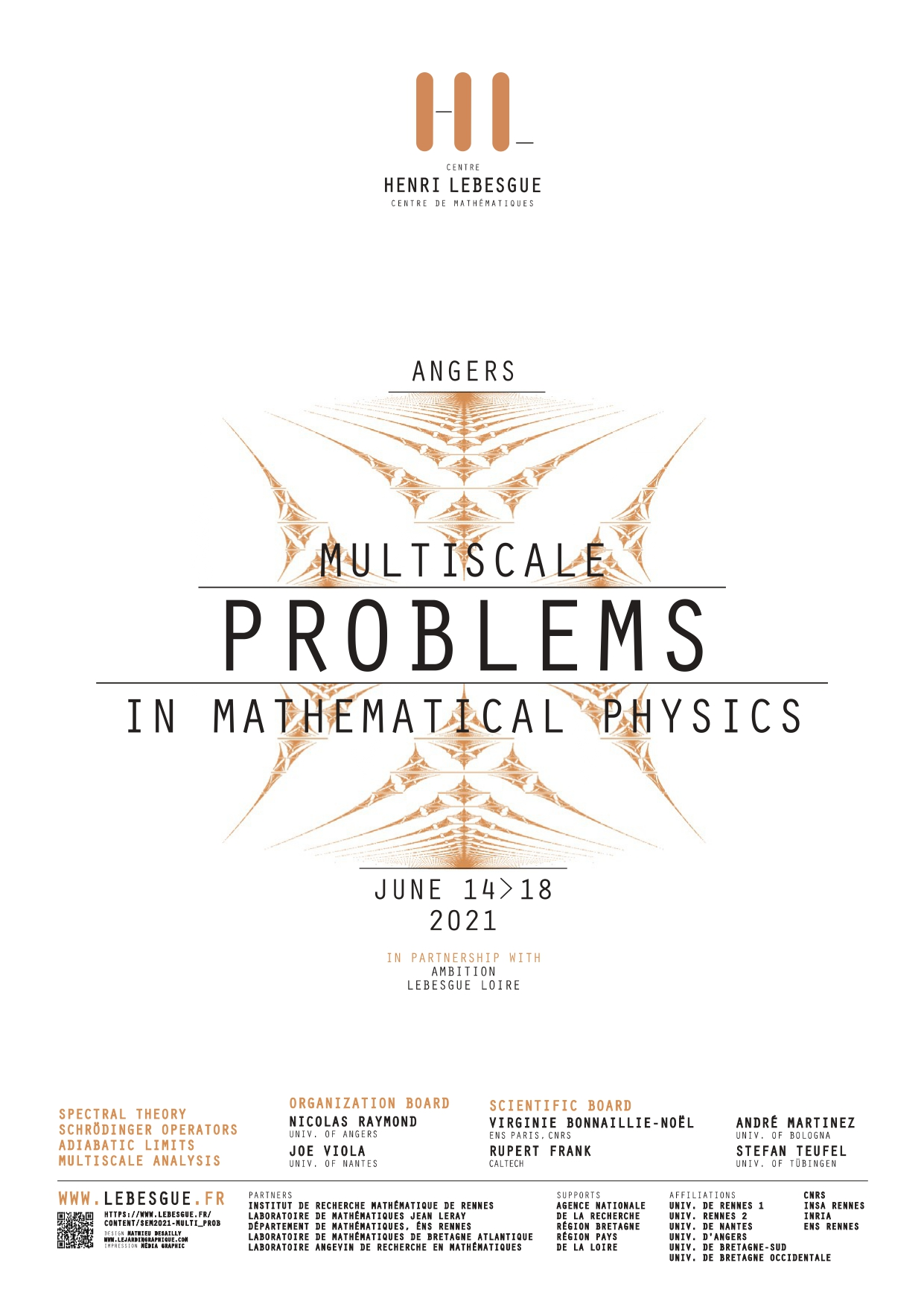placeholder

## Primary tabs

### Monday, June 14, 2021

 All day

Before 01
01
02
03
04
05
06
07
08
09
10
11
12
13
14
15
16
17
18
19
20
21
22
23

Conference - Multiscale problems in mathematical physics
Jun 14, 2021 to Jun 16, 2021Angers, from june 14th to june 16th 2021

Organization board: Nicolas RAYMOND, Joe VIOLA

The aim of this workshop is to present the latest advances involving multiscale phenomena arising in Physics. This will allow for the interaction between different research communities and stimulate new investigations on a large array of recent problems, from dynamical problems to spectral theory.

### Speakers

Paul Alphonse (ENS Lyon)

Victor Arnaiz (Université Paris-Saclay)

Vincent Bruneau (Université de Bordeaux)

Mattia Cafasso (University of Angers)

Éric Cancès (École des Ponts Paristech)

Clotilde Fermanian Kammerer (University Paris-Est Creteil, CNRS)

Louis Garrigue (École des Ponts)

Frédéric Hérau (University of Nantes)

Jonas Lampart (Université de Bourgogne)

Loïc Le Treust (Université d'Aix Marseille)

Léo Morin (Université de Rennes 1)

Tiến-Tài Nguyễn (Université de Paris 13)

Van Hoi Nguyen (Université de Rennes 1)

Nicolas Rougerie (ENS Lyon)

Julien Sabin (École Polytechnique)

Nir Schwartz (Université Paris-Saclay)

Nikolay Tzevtkov (University of Cery-Pontoise)

Conference - Multiscale problems in mathematical physics
Jun 14, 2021

Conference - Multiscale problems in mathematical physics
Jun 14, 2021

In this talk we present a very recent results on the return to the equilibrium for inhomogeneous linearized kinetic equations when the collision kernel has 5 conserved moments (mass, momentum and energy). We study the case when there is an external potential acting on the system of particles. When there is no axisymetry of harmonicity of the potentiel, there is a unique equilibrium state (for a given initial mass and energy), and the return to the equilibrium is the result of a cascade of hypocoercive and damping effects. When there are some axisymmetries or harmonicity of the potential, special solutions appear. We shall try to explain the general scheme of the proof and the main mathematical and physical ideas behind. This a joint work with K. Carrapatoso, J. Dolbeault, S. Mischler, C. Schmeiser and C. Mouhot.

Conference - Multiscale problems in mathematical physics
Jun 14, 2021

Conference - Multiscale problems in mathematical physics
Jun 14, 2021

A central theme in quantum chaos is the study of spectral problems arising in the settings of hyperbolic dynamics. One of the most well known examples for such dynamics is compact hyperbolic manifolds with constant negative curvature, on which one considers the Laplace-Beltrami operator. In our talk we recall a chaotic toy example of this model living on the 2-dimensional torus T2 and called “quantum cat map”. Then we present an analogue of a result originally proved by Dyatlov and Jin in the settings of compact hyperbolic surfaces. Roughly speaking our result means that semiclassical measures, a measure-theoretic invariant of the cat map, cannot concentrate on a proper open set of the torus. The proof relies on semi-classical methods and on the fractal uncertainty principle proved by Bourgain and Dyatlov in 2016.

Conference - Multiscale problems in mathematical physics
Jun 14, 2021

Conference - Multiscale problems in mathematical physics
Jun 14, 2021

La DFT (density functional theory) est la méthode la plus efficace pour modéliser la matière à l'échelle microscopique, elle est extensivement utilisée en physique et chimie quantique à N corps. L'unes des principales questions mathématiques de cette théorie est un problème inverse consistant à trouver un potentiel électrique produisant une certaine densité donnée. Nous introduirons la DFT, ses objectifs, son problème inverse, et le problème ouvert de la convergence aux hautes densités de ce problème inverse.